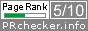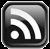# LEARN MS-ACCESS TIPS AND TRICKS

Learn Microsoft Access Advanced Programming Techniques, Tips and Tricks.

### Introduction.

I know your immediate response after looking at the Title will be, "I know all those things, tell me something that I don't know". Well, if you haven't come across the last item (that is an odd one) in the Title before, then that is what I am trying to do here, read on. The first four words are very familiar to us they are Built-in Functions in MS-Access and Worksheet Functions in Excel.

We will catch up with the last one later, after checking the usage of Min() Function (will represent the first four items in the title) in Excel and why we have some difficulty with it in MS-Access when compared with Microsoft Excel.

### Difference between Excel and Access

We are not forgetting the other Functions DCount(), DSum(), DMin(), DMax() and DAvg() of Access at all.

Let us look at the usage of Min() Worksheet Function in Excel. It can find the minimum Value from a Range of Cells in a single Column, from a Row of Cells across Columns or from a Range of Cells spread over several Columns and Rows.

But, when we come back to MS-Access the Min() Function can be used only in a single column (on a single Field) of Data in Query and in Header/Footer Sections of Forms or Reports. Then what do we do to find the Minimum value from more than one Field of data?

Have a look at the sample Table given below to get the gravity of the issue we are in here.

We have received Quotations for Electronic Items from three different Suppliers and we need to know which one is the lowest and from which Supplier? In this case our Min() Function has no use here unless we re-organize the above data into the following format:

To get the required result out this data we need two Queries and we will ignore the duplication of Descriptions, Supplier Names and the Table size in Records etc. for now.

1. Need one Total Query to group on Desc field and the Min() Function to find the minimum Value from the Values Field.
2. Need a second Query, using the first Query and the Table above as Source, JOINed on Desc and MinOfValues Columns of the Total Query with the Desc and Values Fields of the Table to pick all the records from the Table matching with minimum quoted values and Description.

### The ParamArray Method

I consider these steps are excessive work and I know you will agree too. Instead, we can write a User Defined Function with the use of ParamArray and pass the Field Names to the Function and find the Minimum Value from the list. Here is a simple Function with the use of ParamArray declaration to find the Minimum Value from a List of Values passed to it.

```Public Function myMin(ParamArray InputArray() As Variant) As Double
'------------------------------------------------------------------
'Author : a.p.r. pillai
'Date   : November-2008
'URL    : www.msaccesstips.com
'------------------------------------------------------------------
Dim arrayLength As Integer, rtn As Double, j As Integer

'calculate number of elements in Array
arrayLength = UBound(InputArray())

'initialize Null values to 0
For j = 0 To arrayLength
InputArray(j) = Nz(InputArray(j), 0)
Next
'initialize variable with 1st element value
'or if it is zero then a value with high magnitude
rtn = IIf(InputArray(0) = 0, 9999999999#, InputArray(0))

For j = 0 To arrayLength
If InputArray(j) = 0 Then
GoTo nextitem
If InputArray(j) < rtn Then
rtn = InputArray(j)
End If
nextitem:
Next

myMin = rtn
End Function```

Copy and Paste the above Code into a Global Module and save it.

Few simple rules must be kept in mind while writing User Defined Functions using the ParamArray declaration in the Parameter list of the Function.

1. While declaring the Function, the Parameter Variable InputArray() (or any other name you prefer) must be declared with the keyword ParamArray, in place of ByRef or ByVal we normally use to declare parameters to functions.
2. The Data Type must be Variant.
3. The ParamArray declaration must be the last item in the Parameter list if the UDF accepts more than one Parameter.
4. The Optional parameter declarations should not appear before the ParamArray declaration.
5. Since the data type is Variant it can accept any type of values.

With the use of the above myMin() Function we have created a Query on the first Table given above. The SQL and the result image of the Query in Datasheet View are given below.

```SELECT MaterialQuote.Desc,
MaterialQuote.Supplier1,
MaterialQuote.Supplier2,
MaterialQuote.Supplier3,
mymin([supplier1],
[supplier2],
[supplier3]) AS Minimum,
IIf([minimum]=[supplier1],"Supplier1",IIf([minimum]=[supplier2],"Supplier2",IIf([minimum]=[supplier3],"Supplier3",""))) AS Quote
FROM MaterialQuote;```

In the above example we have used only three Field Values to pass to the Function and these can vary depending on your requirement.

### Modified Version of VBA Code

A modified version of the same Function is given below that accepts a Calculation Type value (range 0 to 3) as first Parameter and depending on that we can find Summary, Minimum, Maximum, or Average of values passed to it through the InputArray() Variable.

```Public Function SMMAvg(ByVal calcType As Integer, ParamArray InputArray() As Variant) As Double
'------------------------------------------------------------------------
'calType : 0 = Summary'        : 1 = Minimum
'        : 2 = Maximum'        : 3 = Average
'------------------------------------------------------------------------
'Author  : a.p.r. pillai'Date    : November 2008
'URL     : www.msaccesstips.com
'------------------------------------------------------------------------
Dim rtn, j As Integer, arrayLength As Integer
Dim NewValue As Variant

On Error GoTo SMMAvg_Err

If calcType < 0 Or calcType > 3 Then
MsgBox "Valid calcType Values 0 - 3 only", , "SMMAvg()"
Exit Function
End If

arrayLength = UBound(InputArray())
For j = 0 To arrayLength
InputArray(j) = Nz(InputArray(j), 0)
Next

Select Case calcType
Case 1
rtn = InputArray(0)
rtn = IIf(rtn = 0, 9999999999#, rtn)
Case 2
rtn = InputArray(0)
Case Else
rtn = 0
End Select

For j = 0 To arrayLength
NewValue = InputArray(j)
If NewValue = 0 Then
GoTo nextitem
Select Case calcType
Case 0, 3
rtn = rtn + NewValue
Case 1
rtn = IIf(NewValue < rtn, NewValue, rtn)
Case 2
rtn = IIf(NewValue > rtn, NewValue, rtn)
End Select
nextitem:
Next

If calcType = 3 Then
rtn = rtn / (arrayLength + 1)
End If

SMMAvg = rtn

SMMAvg_Exit:
Exit Function

SMMAvg_Err:
MsgBox Err.Description, , "SMMAVG()"
SMMAvg = 0
Resume SMMAvg_Exit
End Function
```

The Function name was defined using the first letters of the Calculation Types that the Function can perform and I hope you like it too.

When any of the values in the InputArray() element is Zero then that is ignored and will not be taken as minimum value.

We can use this Function in Text Boxes on Forms or Reports by passing Values from other Controls. Use it at your own risk.

Share:

Comments subject to moderation before publishing.

## TranslateSubscribe in a readerYour email address:

Delivered by FeedBurner